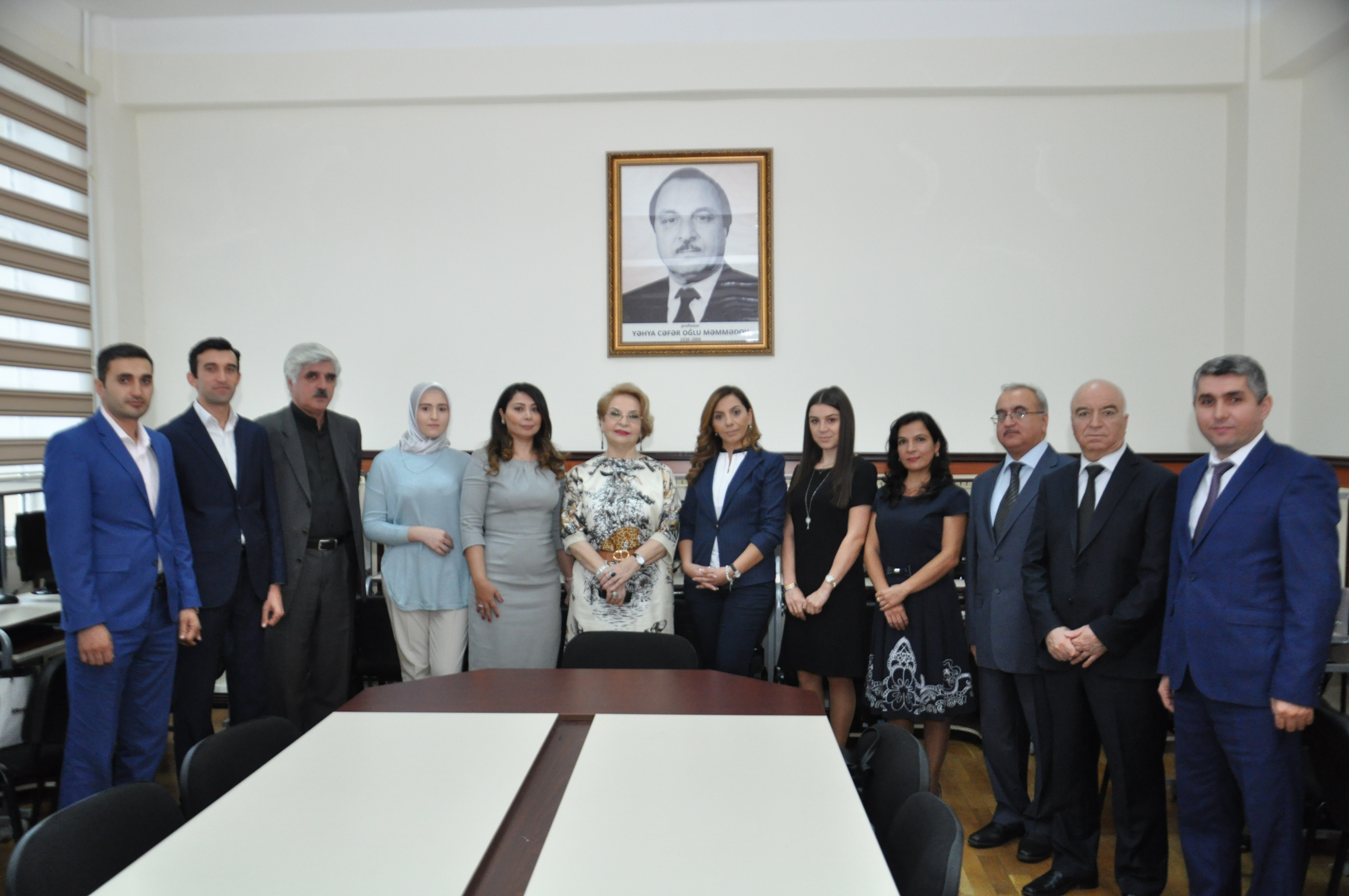﻿ BAKU STATE UNIVERSITY

## The chair of Computational MathematicsThe chair of Computational Mathematics was founded in 1961. First chief of the chair was prof. A.S.Habibzadeh. Since 1965 to 2000 the chief of the chair was prof. Yahya Mammadov – an Honored Science Worker, State Prize Laureate, and corresponding member of NASA. Computational Mathematics was developed as a detached scientific direction owing to colossal work of prof. Y. Mammadov.

At present, the staff of the chair is as following: d.p.-m.s., prof. G.Y.Mehdiyeva (chief of the chair), d.p.-m.s., prof. V.R.Ibrahimov, c.p.-m.s., assoc.prof. A.Y.Aliyev, c.p.-m.s., assoc.prof. Z.B.Seyidov,  senior lecturer B.B.Balakishiyev, c.p.-m.s., assoc.prof. E.I.Azizbayov, assoc.prof. ph.d M.N.Imanova, c.p.-m.s, lecturer,  G.X.Shafiyeva, lecturer, ph.d A.M.Guliyeva, senior laboratory assistant R.R.Mirzayev, senior laboratory assistant G.A.Agayeva, laboratory assistant B.Y.Shakhova.

The staff of the chair conducts lectures on the disciplines of computer and programming, bases of computer sciences, the discrete mathematics, a practical work on the computer, methods of calculations, introduction in algorithmic languages, calculus mathematics for bachelor students and the disciplines of computer technologies in science and education, the decision of the operational equations with approximate methods, the decision of the algebraic equations with approximate methods, some questions of computer science, the decision of the differential equations in partial derivatives with approximate methods, the role of the approximate methods in the decision of problems of  natural sciences, approximate methods of the regular differential equations, spline-function and their application, incorrect problems, decision of variation problems with numerical methods.

By staff of the chair were published more than 500 scientific articles, 7 monographs, 3 manuals, 15 tutorials.

Under the direction of corresponding member of NАSА, prof. Y.J. Mammadov, prof. A.A.Dosiyev, prof. V.R.Ibrahimov, prof. F.A.Tagiyev, prof. A.I.Hasanov and prof. G.M.Amiraliyev were defended more than 50 Ph.D theses.

Scientific work of the chair is conducted in following directions: the numerical, analytically-approximated methods of the decision of the ordinary differential equations, the differential equations in the partial derivative and Volterr- type integral equations.

Employments:

Mehdiyeva G.Y. - Chief of the chair, professor, doctor of physical and mathematical sciences

Ibrahimov V.R.- professor, doctor of physical and mathematical sciences

Seyidov Z.B. - associate professor, candidate of physical and mathematical sciences

Aliyev A.Y. - associate professor, candidate of physical and mathematical sciences

Gurbanov I.A. - associate professor, candidate of physical and mathematical sciences

Balakishiyev B.B. -senior lecturer

Azizbayov E.I.- associate professor, candidate of physical and mathematical sciences,
a laboratory CEO on the Computational Mathematics

M.N.Imanova -assoc.prof. ph.d

G.X.Shafiyeva-c.p.-m.s, lecturer

Guliyeva A.M.-  ph.d,lecturer .

R.R.Mirzayev- senior laboratory assistant.

G.A.Agayeva-senior laboratory assistant.

B.Y.Shakhova- laboratory assistant.